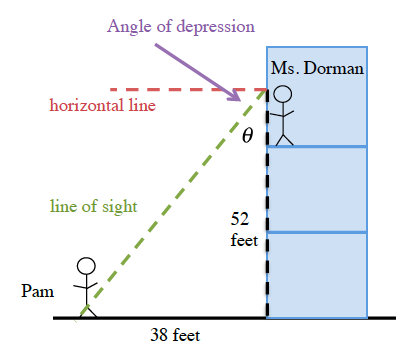### Home > INT2 > Chapter 8 > Lesson 8.1.1 > Problem8-9

8-9.

As Ms. Dorman looks from the window of her third-story classroom, she notices Pam in the courtyard. Ms. Dorman’s eyes are $52$ feet above ground and Pam is $38$ feet from the building. Draw a diagram of this situation. What is the angle at which Ms. Dorman has to look down; that is, what is the angle of depression? (Assume that Ms. Dorman is looking at the spot on the ground below Pam.)

Start by drawing a detailed diagram showing Pam, Ms. Dorman, and the building. Label completely. Remember that the angle of depression is the angle between Ms. Dorman's line of sight and the horizontal line, not between her line of sight and the building.Use the tangent ratio to solve for $θ$. $θ$ is the adjacent angle to angle of depression.

$\tan(\theta)=\frac{38}{52}$

$\theta=\tan^{-1}\left(\frac{38}{52}\right)\approx36.16^\circ$

Since $θ +$ angle of depression $= 90^\circ$, the angle of depression is $≈ 53.84^\circ$.